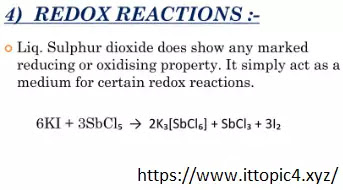# Redox Reactions in liquid SO2, Solvolysis Reaction in liquid SO2 And Metathetical Reaction in liquid SO2

1. ## Redox Reactions in liquid SO2:

Redox reaction is actually oxidation reaction of compound took place in a reaction.## Redox Reactions in liquid SO2

1. 6KI + 3SbCl5SbCl3 + 2K3[SbCl]

In this reaction six molecules of KI potassium iodide react with three molecules of SbCl5 antimony penta chloride (Sb = stibium) to produce the product SbCl3 antimony trichloride and a complex of potassium antimony hexa chloride and two molecules of I2.

In this reaction in reactant KI the oxidation number of Iodine I is (-1) and in product I2 (diatomic iodide) its oxidation number is (0) so here oxidation number increase from (-1 to 0) so oxidation took place in same in SbCl5 the oxidation of Sb is +5 in reactant and the oxidation of SbCl3 is Sb= +3 so here oxidation number decrease from (5 to 3) so reduction is redox place. This reaction is redox reaction in the presence of liquid SO2 and is reversible reaction in which Iodide undergoes in oxidation and antimony undergoes in reduction.

1. 5R2SO3 + I2R2SO4 + 2RI + SO2

(S= +4)      (I= 0)           (S= +6)     (I= -1)

In this reaction the oxidation state of R2SO3 is increasing from (S= +4 to +6) and oxidation state of I2 iodine is decreasing from (I= 0 to -1), they both react to produce the product R2SO4 and a 2RI and a molecules of SO2 in the presence of liq. SO2.

1. 5SbCl5 + 6KI3I2 + SbCl3 + 2K3[SbCl6]
(Sb= +5)   (I= 1)             (I= 0)    (Sb= +3)    (Sb= +3)

This reaction the oxidation state of SbCl5 is decreasing from (Sb= +5 to +3) and oxidation state of KI2 potassium iodide is also decreasing from (KI= 1 to 0), they both react to produce the product SbCl3 and a 3I2 and a molecules of 2K3[SbCl6] in the presence of liq. SO2.

1. 2FeCl3 + 2KI2FeCl2 + I2 + 2KCl

(Fe= +3)    (I= -1)             (Fe= +2)   (I= 0)

In this reaction the oxidation state of FeCl3 is decreasing from (Fe= +3 to +2) and oxidation state of KI potassium iodide is increasing from (KI= -1 to 0), they both react to produce the product FeCl2 and a I2 and a molecules of 2KCl in the presence of liq. SO2.

1. ## Solvolysis Reaction in liquid SO2:

Solvo means solvent and lysis means breakdown. It is a solvolytic reaction.

1. NaOH + 2NH3NaNH2 + NH4OH

Here NH3 ammonia is breaking down because it is solvolysis (solvent breaking) reaction so NH3is breaking down to produce NaNH2 in product. Reaction produced in the presence of liquid SO2.

1. PCl5 + SO2POCl3 + SOCl2

This reaction also shows the breaking down of SO2 in to SO in the presence of aqueous Sulphur dioxide.

1. AsCl5 + SO2AsOCl3 + SOCl2

Here same the solvolysis of SO2 is occurring in the presence of liquid SO2 in which SO2 is solvolyzed into SOCl2.

Here are some covalent halides like PCl5, UCl6, WCl6 undergo solvolysis reactions in liq. SO2 and form oxyhalides. In all these reactions the concentration of SO2+ ion is increased.

1. PBr5 + SO2(liq)       POBr3 + SOBr22. WCl6 + SO2(liq)                WOCl4 +SOCl23. NbCl5 + 2SO2(liq.)              NbOCl3 + SOCl24. Zn(C2H5)2 +2SO2(liq.)               ZnSO3 + SO(C2H5)25. UCl6 + 2SO2(liq.)                     UO2Cl2 + 2SOCl21. ## Metathetical Reaction in liquid SO2:

1. 2CH3COOAg + SOCl22AgCl + SO[CH3COOH]2

Siver salt of acetic acid CH3COOAg react with SOCl2 in the presence of liquid Sulphur dioxide to produce AgCl and SO[CH3COO]2  sulphate acetic acid. This reaction is called metathetical reaction because here AgCl  is precipitated out because if we put AgCl what it will be soluble and same AgCl  liquid SO2  it will be insoluble so it will precipitate out in the reaction.

1. 2KBr + SOCl22KCl + SOBr2

In this reaction two molecules of potassium chloride KCl will precipitate out when KCl is dissolved in liquid Sulphur dioxide.